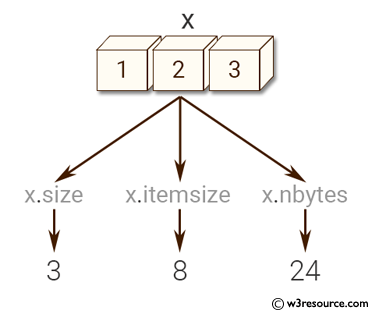﻿ NumPy: Find the number of elements of an array, length of one array element in bytes - w3resource# NumPy: Find the number of elements of an array, length of one array element in bytes

## NumPy: Array Object Exercise-16 with Solution

Write a NumPy program to find the number of elements of an array, length of one array element in bytes and total bytes consumed by the elements

Pictorial Presentation:Sample Solution:-

Python Code:

``````import numpy as np
x = np.array([1,2,3], dtype=np.float64)
print("Size of the array: ", x.size)
print("Length of one array element in bytes: ", x.itemsize)
print("Total bytes consumed by the elements of the array: ", x.nbytes)
```
```

Sample Output:

```Size of the array:  3
Length of one array element in bytes:  8
Total bytes consumed by the elements of the array:  24
```

Python Code Editor:

Have another way to solve this solution? Contribute your code (and comments) through Disqus.

What is the difficulty level of this exercise?

Test your Python skills with w3resource's quiz

﻿

## Python: Tips of the Day

Free up Memory:

• Manual garbage collection can be performed on timely or event based mechanism.
```import gc
collected_objects = gc.collect()
```